# How to calculate Excess fiber length due to stranding in a fiber optic cable

The method to calculate the excess fiber length in a stranded loose tube fiber optic cable is very easy. The formula is nothing but our old Pythagoras formula.

In helical stranding, the elements form a screw line which may look like a spiral staircase. The length of pitch of this spiral screw line after a full turn of 360° is commonly known in cable industry as Lay length or simply Pitch. The word ‘Pitch’ is common at some countries, where as in some other parts of the world, it is Lay length. The concept is same.

The important thing to keep in mind is, the excess length what we are discussing here is different from the excess fiber length inside the loose tube. Here we are talking about the loose tube excess length due to stranding, and thereby of course the fiber length. The excess fiber length in loose tube is assumed to be zero after the loose tubes are paid off from the pay off station in stranding machine.

The angle between the stranding elements and the cable cross section is known as the slope of stranding or stranding angle a (alpha in formula).

The distance between the axis of the cable core and the middle of the stranding element is termed as the Stranding radius, denoted by R. Look the diagram below. The R is self explanatory in this diagram.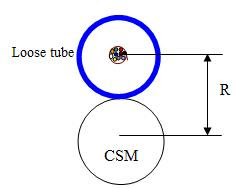Therefore, the excess tube length (or fiber) L of a stranding element and the Stranding angle a (alpha in formula) can be expressed as: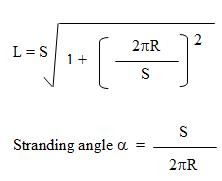Where,
R = Standing radius in mm
L = Length of stranding element in mm
S = Lay length in mm
2 pi R = Circumference of the stranding circle.

And because of this stranding, the elements or tubes will be longer in length than the CSM (generally FRP in a nonmetallic cable). This excess length due to stranding in percentage can be expressed by the following formula: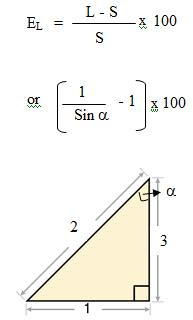The screw line is a three dimensional curve, and because of that the radius of curvature or commonly known in cable industry as bending radius can be calculated by the following formula: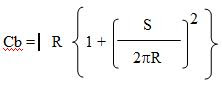Cb = bending radius of cable in mm. The above formulas are important for a cable design engineer to calculate the excess fiber length and bending radius of the cable. For the strength and attenuation performance of an optical fiber, the cable should not be bent too sharply. A measure of it is the minimum permissible radius Cb. A typical value for standard fibers is Cb = 50 mm. If the bending radius Cb and the stranding radius R are known, then the Lay length can be calculated from the following: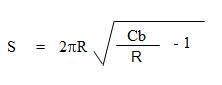QuestTel shall have no liability for any error or damage of any kind resulting from the use of this document.